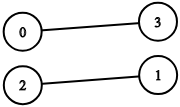## #StackBounty: #machine-learning #neural-networks #optimization #approximation #polynomial Quantifying the universal approximation theorem

### Bounty: 100

Let $$mgeq 1$$ be an integer and $$Fin mathbb{R}[x_1, dots, x_m]$$ be a polynomial. I want to approximate $$F$$ on the unit hypercube $$[0, 1]^m$$ by a (possibly multilayer) feedforward neural network. The activation function is $$mathrm{tanh}$$ for all the connections.

Let $$varepsilon>0$$ be a real number. If I want the approximation to deviate from $$F$$ by less than $$varepsilon$$ in the $$L^2$$ norm what is the smallest possible number of non-zero weights?

It is kind of stupid to approximate a function that is known to be polynomial by a neural network but I just wanted to get more quantitative insight into the universal approximation theorem (and polynomials seem to be the most accessible class of functions).

Get this bounty!!!

## #StackBounty: #algorithms #optimization #approximation #scheduling #intervals 2D interval scheduling problem

### Bounty: 50

Suppose I give you $$n$$ axis-aligned rectangles with a specified width, height, and x-position (of the left edge) $${(w_i, h_i, x_i) mid i in {0, ldots, n – 1}}$$, as well as a bound $$(y_mathrm{min}, y_mathrm{max})$$ on the valid y-positions. These rectangles must have integer-valued coordinates for their vertices. Is there an efficient (e.g.: $$O(n^3)$$) approximation algorithm that gives a sequence $${y_0, ldots, y_{n – 1}}$$ of y-positions such that the number of overlaps between any two rectangles (i.e.: edges in the overlap graph) is minimized (and hopefully zero)?

Note that if $$y_mathrm{max} = 10$$ and $$h_k = 3$$, then $$y_k = 9$$ would be invalid, since the top edge would be at $$12$$ which is more than $$10$$.

Get this bounty!!!

## #StackBounty: #algorithms #graphs #approximation #vertex-cover Vertex cover of bipartite graph

### Bounty: 50

A vertex cover is a set of vertices such that each edge of the graph is incident to at least one vertex of the set.
A minimum vertex cover is a vertex cover with minimal cardinality.

https://codeforces.com/blog/entry/63164

Consider a set of all minimum vertex covers of a given bipartite graph,
our task is to divide all vertices of the graph into three sets.

A vertex is in set

• N (“Never”) if there is no minimum vertex cover containing this vertex.
• A (“Always”) if it is a part of every minimum vertex cover of the given graph.
• E (“Exists”), otherwise.

I don’t understand the variables. I have an edge from maximum matching then I might have both the endpoints of that edge in the vertex cover. But this scheme does not allow this.

This is the graph I have ($$2K_2$$) two edges in the maximum matching:For the first edge I took left vertex in the cover
$$x_1=0$$ (according to the blog in the link)
for the second edge I took right vertex in the cover $$x_2=1$$.

Questions:

1. How final 2-sat will be true?
2. Can anyone explain what will be the relation between x1 and x2 ?

Get this bounty!!!